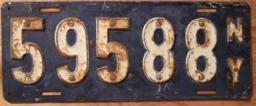# Property 4047

Seven times the number minus 3 is as big as three times the same number minus 7. Which number has this property?

x =  2.5

### Step-by-step explanation:

7x-3 = 3x+7

7·x-3 = 3·x+7

4x = 10

x = 10/4 = 2.5

x = 5/2 = 2.5

Our simple equation calculator calculates it.Did you find an error or inaccuracy? Feel free to write us. Thank you!

Tips for related online calculators
Do you have a linear equation or system of equations and looking for its solution? Or do you have a quadratic equation?